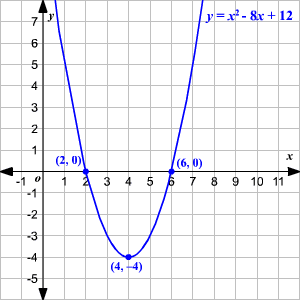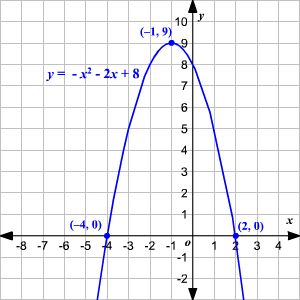# Graphing Quadratic Equations Using Factoring

A quadratic equation is a polynomial equation of degree $2$ .  The standard form of a quadratic equation is

$0=a{x}^{2}+bx+c$

where   $a,b$ and $c$ are all real numbers and $a\ne 0$ .

If we replace $0$ with $y$ , then we get a quadratic function

$y=a{x}^{2}+bx+c$

whose graph will be a parabola .

The points where the graph intersects the $x$ -axis will be the solutions to the equation, $a{x}^{2}+bx+c=0$ . That is, if the polynomial $a{x}^{2}+bx+c$ can be factored to $\left(x-p\right)\left(x-q\right)$ , we know by the zero product property that if $\left(x-p\right)\left(x-q\right)$ = 0 , either $\left(x-p\right)=0$ or $\left(x-q\right)=0$ . Then $p$ and $q$ are the solutions to the equation $a{x}^{2}+bx+c=0$ and therefore the $x$ -intercepts of the quadratic equation.

Since the $x$ -coordinate of the vertex of a parabola is exactly the midpoint of the $x$ -intercepts , the $x$ -coordinate of the vertex will be $\frac{p\text{\hspace{0.17em}}+\text{\hspace{0.17em}}q}{2}$ .

You can use the $x$ -coordinate of the vertex to find the $y$ -coordinate.

Now you have the vertex and $2$ other points on the parabola (namely, the $x$ -intercepts). You can use these three points to sketch the graph.

Example 1:

Graph the function $y={x}^{2}-8x+12$ using factoring.

Compare the equation with the standard form, $y=a{x}^{2}+bx+c$ . Since the value of $a$ is positive, the parabola opens up.

Factor the trinomial, ${x}^{2}-8x+12$ . Identify $2$ numbers whose sum is $-8$ and the product is $12$ . The numbers are $-2$ and $-6$ . That is, ${x}^{2}-8x+12=\left(x-2\right)\left(x-6\right)$ .

${x}^{2}-8x+12=0⇒\left(x-2\right)\left(x-6\right)=0$

So, by the zero product property, either $\left(x-2\right)=0$ or $\left(x-6\right)=0$ . Then the roots of the equation are $2$ and $6$ .

Therefore, the $x$ -intercepts of the function are $6$ and $2$ .

The $x$ -coordinate of the vertex is the midpoint of the x -intercepts. So, here the $x$ -coordinate of the vertex will be $\frac{2\text{\hspace{0.17em}}+\text{\hspace{0.17em}}6}{2}=4$ .

Substitute $x=4$ in the equation $y={x}^{2}-8x+12$ to find the $y$ -coordinate of the vertex.

$\begin{array}{l}y={\left(4\right)}^{2}-8\left(4\right)+12\\ \text{\hspace{0.17em}}\text{\hspace{0.17em}}\text{\hspace{0.17em}}\text{\hspace{0.17em}}=16-32+12\\ \text{\hspace{0.17em}}\text{\hspace{0.17em}}\text{\hspace{0.17em}}\text{\hspace{0.17em}}=-4\end{array}$

That is, the coordinates of the vertex are $\left(4,-4\right)$ .

Now we have 3 points $\left(4,-4\right)$ , $\left(2,0\right)$ and $\left(6,0\right)$ which are on the parabola. Plot the points. Join them by a smooth curve and extend the parabola.Example 2:

Graph the function $y=-{x}^{2}-2x+8$ using factoring.

Compare the equation with the standard form, $y=a{x}^{2}+bx+c$ . Since the value of $a$ is positive, the parabola opens up.

Factor the trinomial, $-{x}^{2}-2x+8$ .

First, factor out $-1$ .

$-{x}^{2}-2x+8=-1\left({x}^{2}+2x-8\right)$

Factor the expression in the parenthesis. Identify $2$ numbers whose sum is $2$ and the product is $-8$ . The numbers are $4$ and $-2$ . That is, ${x}^{2}+2x-8=\left(x+4\right)\left(x-2\right)$ .

Then, the given function becomes $y=-\left(x+4\right)\left(x-2\right)$ .

So, $y=0$ implies, by the zero product property, $x+4=0$ or $x-2=0$ .

Therefore, the $x$ -intercepts of the graph are $-4$ and $2$ .

The $x$ -coordinate of the vertex of a parabola is the midpoint of the $x$ -intercepts. So, here the $x$ -coordinate of the vertex will be $\frac{-4\text{\hspace{0.17em}}+\text{\hspace{0.17em}}2}{2}=-1$ .

Substitute $x=-1$ in the equation $y=-{x}^{2}-2x+8$ to find the $y$ -coordinate of the vertex.

$\begin{array}{l}y=-{\left(-1\right)}^{2}-2\left(-1\right)+8\\ \text{\hspace{0.17em}}\text{\hspace{0.17em}}\text{\hspace{0.17em}}\text{\hspace{0.17em}}=-1+2+8\\ \text{\hspace{0.17em}}\text{\hspace{0.17em}}\text{\hspace{0.17em}}\text{\hspace{0.17em}}=9\end{array}$

So, the coordinates of the vertex are $\left(-1,9\right)$ .

Now we have $3$ points $\left(-1,9\right)$ , $\left(-4,0\right)$ and $\left(2,0\right)$ which are on the parabola. Plot the points. Join them by a smooth curve and extend the parabola.